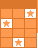Challenge Board: Score points by answering questions correctly.# Algebra Review

Gain points by answering the questions correctly. Lose points for incorrect answers. Categories: number operations(order of operations), solving equations(mult-step and all variable), systems of equations, graphing linear equations, factoring, expanding, square roots (simplifying perfect and non-perfect sq roots as well as multiplying and adding) and exponents (negative and zero).This activity was created by a Quia Web subscriber. Learn more about QuiaCreate your own activities